## 10.3 Variation with Time of Energy in Simple Harmonic Motion JavaScript HTML5 Applet Simulation Model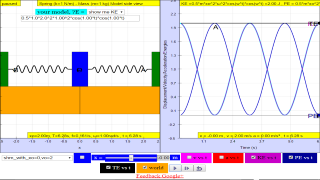SHM17

### 1.3 a)    Variation with time of energy in simple harmonic motion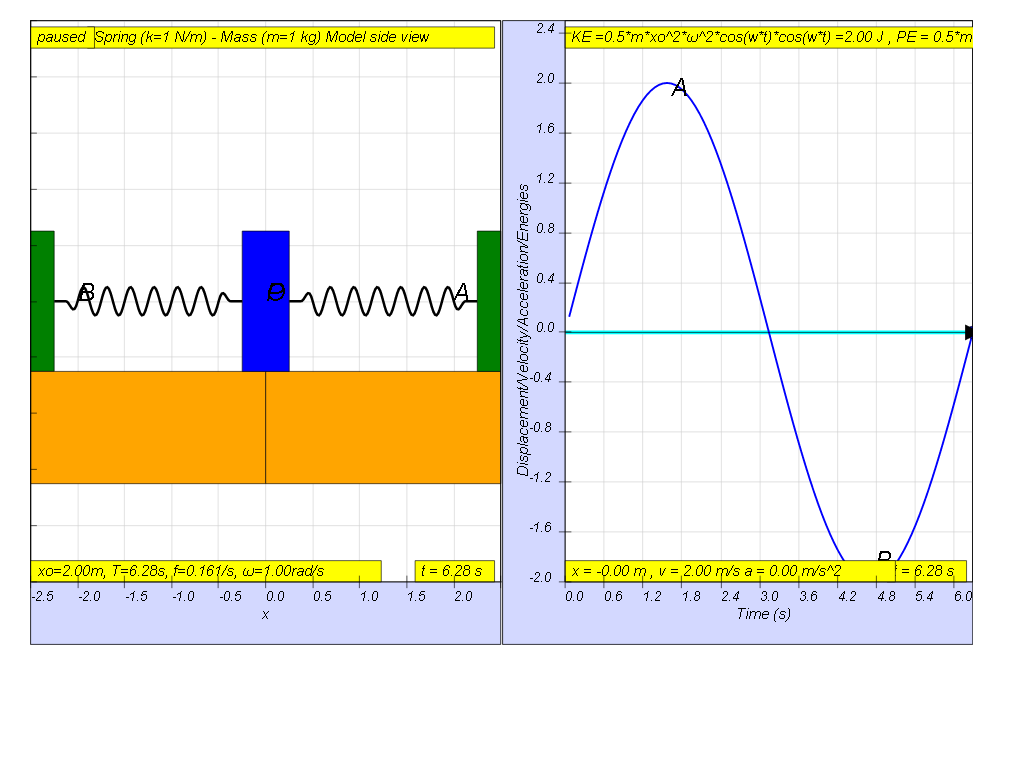If the variation with time of displacement is as shown, then the energies should be drawn as shown.

recalling Energy formula

KE = ½ m v2

PE =  ½ k x2

in terms of time t,

x = x0 sin(ωt)

differentiating with t gives

v = v0 cos (ωt)

therefore, KE = ½ m v2= ½ m (v0 cos (ωt))2= ½ m (x02ω2)cos (ωt))2

similarly

PE = ½ k x2= ½ (mω2 )(x0 sin (ωt))2= ½ m (x02ω2 )sin (ωt))2

therefore total energy is a constant value in the absence of energy loss due to drag (resistance)

TE = KE + PE = ½ m (x02ω2 )[cos2(ωt) + sin2(ωt))] = ½ m (x02ω2)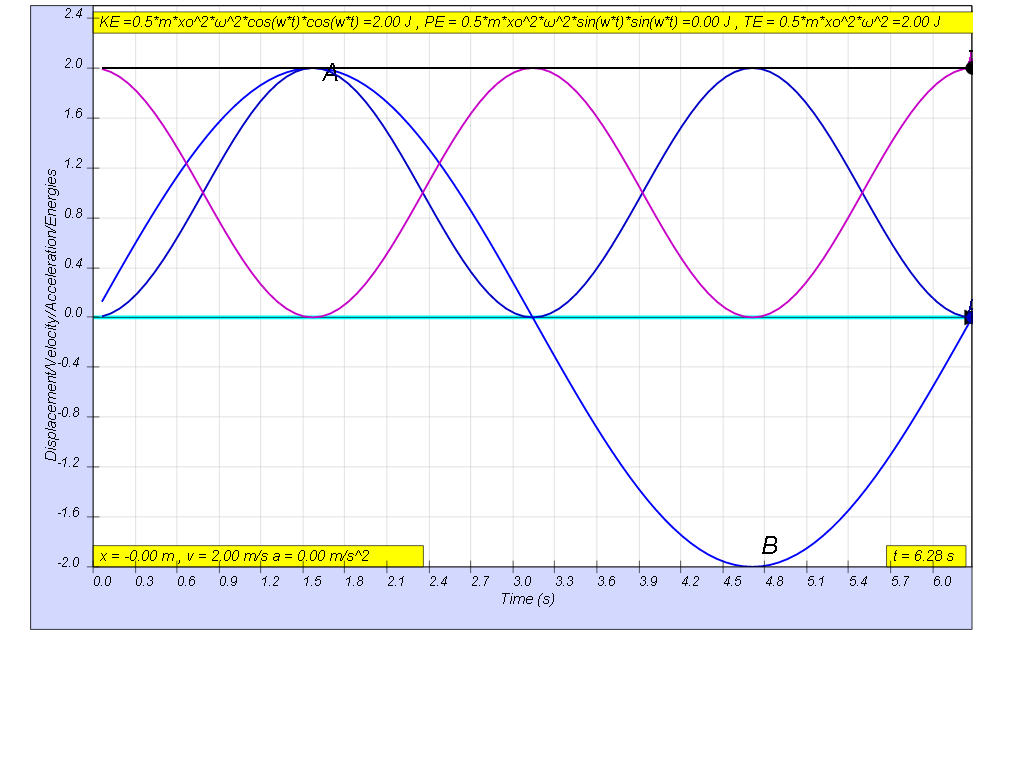this is how the x vs t looks together of the energy vs t graphs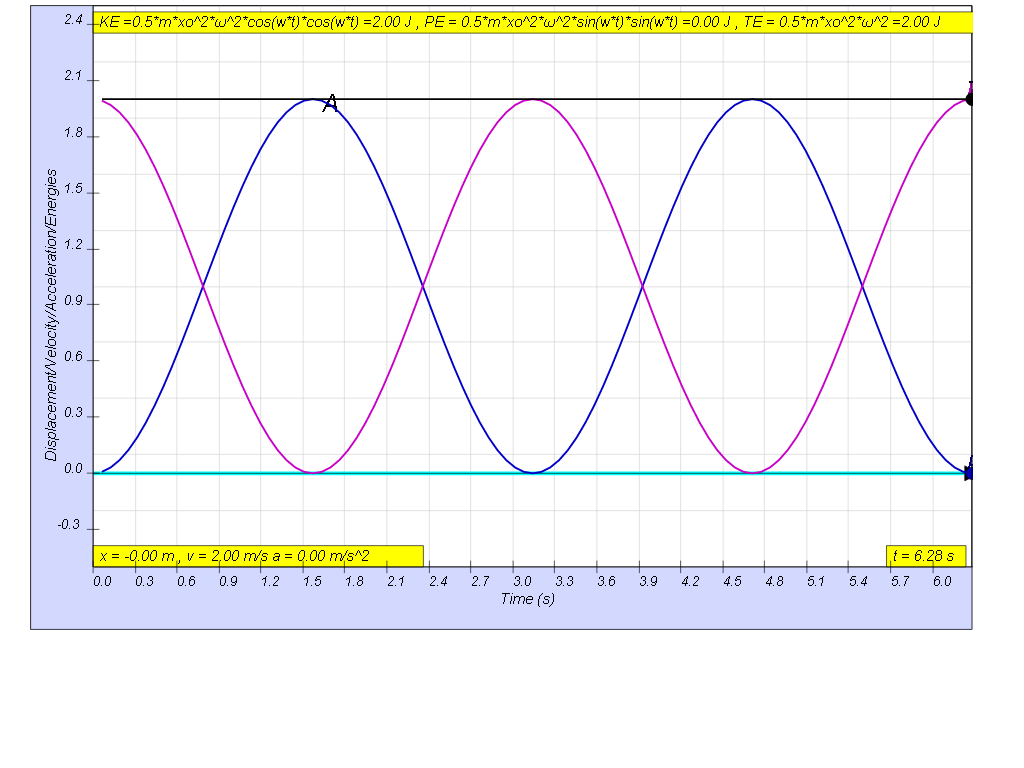### 1.3.1 Summary

the table shows some of the common values
 general energy formula SHM energy formula when t = 0 when t = T/4 when t = T/2 when t = 3T/4 when t = T KE = ½ m v2 ½ m (x02ω2)cos (ωt))2 ½ m (x02ω2) 0 ½ m (x02ω2) 0 ½ m (x02ω2) PE =  ½ k x2 ½ m (x02ω2)cos (ωt))2 0 ½ m (x02ω2) 0 ½ m (x02ω2) 0 TE = KE + PE TE = ½ m (x02ω2) ½ m (x02ω2) ½ m (x02ω2) ½ m (x02ω2) ½ m (x02ω2) ½ m (x02ω2)

### Translations

Code Language Translator Run### Software Requirements

SoftwareRequirements

 Android iOS Windows MacOS with best with Chrome Chrome Chrome Chrome support full-screen? Yes. Chrome/Opera No. Firefox/ Samsung Internet Not yet Yes Yes cannot work on some mobile browser that don't understand JavaScript such as..... cannot work on Internet Explorer 9 and below

### CreditsThis email address is being protected from spambots. You need JavaScript enabled to view it.

### end faq

http://iwant2study.org/lookangejss/02_newtonianmechanics_8oscillations/ejss_model_SHM17/SHM17_Simulation.xhtml

## Apps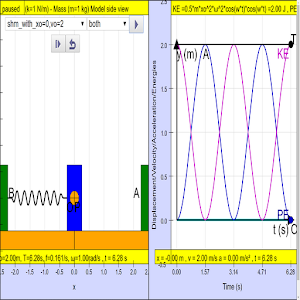https://play.google.com/store/apps/details?id=com.ionicframework.shm17app307408&hl=en

Other resources

https://ggbm.at/pY4Hvugh